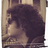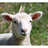Welcome to our new forum
All users of the legacy CODESYS Forums, please create a new account at account.codesys.com. But make sure to use the same E-Mail address as in the old Forum. Then your posts will be matched.

## Codesys writing csv-file to Raspberry/ RPi directory document.SUBSCRIPTION_OPTIONS = { "thing": "topic", "subscribed": false, "url": "subscribe", "icon": { "css": "fa fa-envelope-o" } };

SpeedyG
2018-08-14
2021-06-07
•SpeedyG - 2018-08-14

Hi there, (English and German are alright)

I am feeling like I am missing something small, but I still cannot find it:
I am doing an internship and have to solve the problem asap (almost at the end). It would be a big relieve if you could help me! Here the problem:

I am using Codesys V3.5 SP11 Patch 3+ and I am working with Codesys Control for RPi

My aim is to create a csv-file in Codesys and save it on the sd card from the RPi. To make things easier I have two programs running, one on
1) Codesys Control Win V3 and one on
2) Codesys Control for RPi.
The code is the same (very simplified), only the machine and directory are different.
The program is working on the Windows machine. It is creating the file and writing in it the information I give it in the code. On the RPi however the program is running (no Error?!), but it isn't writing a file. My thinking was that it has something to do with the directory, but after researching over one week I still haven't found the right information.

I have attached the program and the subprogram so you can have a look on it. (not all variables are used bc I shortened the program)

One of the directories I tried is: 'cd \home\pi\Downloads\test.csv' (probably totally wrong)

Thank you so much for your help!!
SpeedyG

VAR       bWrite         BOOL
VAR      pEnd         POINTER TO STRING
VAR      resultdata_csv      ARRAY[1..400000] OF BYTE
VAR      handleFile      DWORD
VAR      dwWritten      DWORD
VAR      szSeperator      STRING(1)            ';'
VAR      iCounter1      INT
VAR      Copy_of_bWrite      BOOL            TRUE
VAR      FilePathAndName      String            'cd \home\pi\Downloads\test.csv
VAR      CRLF         STRING(2)         '$R$N'
VAR      LF         String(1)         '$N' VAR Copy_of_resultdata_csv ARRAY[1..400000] OF BYTE VAR big DINT VAR i DINT VAR z DINT VAR zahl REAL VAR Copy_of_pEnd POINTER TO STRING VAR fopen FILE.Open VAR fwrite file.Write VAR fclose file.Close (* ---------------------------- Fill Head Data -------------------------------- *) IF bWrite THEN pEnd := ADR(resultdata_csv); pEnd := StrCatPtr('987654321', pEnd); fopen( xExecute:= TRUE, xDone=> , xBusy=> , xError=> , sFileName:= FilePathAndName, eFileMode:= 0, xExclusive:= , eError=> , hFile=> handleFile); fwrite( xExecute:= fopen.xDone, xAbort:= , udiTimeOut:= , xDone=> , xBusy=> , xError=> , xAborted=> , hFile:= handleFile, pBuffer:= ADR(resultdata_csv), szSize:= pEnd - ADR(resultdata_csv) , eError=> ); fclose( xExecute:= fwrite.xDone, xDone=> , xBusy=> , xError=> , hFile:= handleFile, eError=> ); IF fclose.xDone THEN bWrite:= FALSE; END_IF END_IF  StrCatPtr (FUN).txt [320 Bytes] PLC_PRG (PRG)_short.txt [1.37 KiB] •johnlee - 2018-08-27 cd \home\pi\Downloads\test.csv -> /home/pi/Downloads/test.csv •SpeedyG - 2018-08-28 Ouch, that hurts. I guess it was too obvious. -.- Thank you @johnlee. •galexis - 2021-06-01 I try to use your code, but it work only one time. I can't to write a other line on csv: csv doesn't change. I need to clean code and reload code on Rpi: it works, but first line of csv is replace. What is the problem ? Thank for your help. •Ingo - 2021-06-03 Didn't try it, but i expect two problems from the code: 1st: fopen( xExecute:= TRUE,  xExecute is not toggled, so it is only executed once. maybe this would help: fopen( xExecute:= bWrite,  2nd: eFileMode:= 0,  if you want to append a line, you need to set the filemode to "append". But this was just the first impression, on first sight. Cheers, Ingo •galexis - 2021-06-03 Hi, yes, if file already exist : efilemode had to be 3 and if it doesn't exist efilemode had to be 0. I see fopen.xExecute... •galexis - 2021-06-03 I do: PROGRAM POU_RPi VAR bWrite: BOOL; pEnd: POINTER TO STRING; resultdata_csv: ARRAY[1..400000] OF BYTE; Hfile: DWORD; //szSeperator: STRING(1):=';'; FilePathAndName: STRING:= '/home/pi/Downloads/test.csv'; CRLF: STRING(2):='$R$N'; LF: STRING(1):= '$N';
fopen: FILE.Open;
fopenadd: FILE.Open;
fwrite: file.Write;
fclose: file.Close;
test: INT :=1;
NumAction: INT;
code_erreur: INT;
END_VAR

(* ---------------------------- Ecrire les data dans le CSV-------------------------------- *)

IF bWrite THEN

pEnd := ADR(resultdata_csv);
pEnd := StrCatPtr('Test n°', pEnd);
pEnd := StrCatPtr(INT_TO_STRING(test), pEnd);
pEnd := StrCatPtr(Lf, pEnd);
//pEnd := StrCatPtr(CRLF, pEnd);

CASE NumAction OF
0: ;

1:  (*Ouvrir le fichier*)
//en mode ajout
fopenadd.sFileName:=FilePathAndName;
fopenadd.eFileMode:=FILE.MODE.MAPPD;
fopenadd.xExclusive:=TRUE;
fopenadd( xExecute:=TRUE);
//Le fichier existe
IF fopenadd.xDone THEN
Hfile:=fopen.hFile;
NumAction:=2;
END_IF
//Erreur
IF fopenadd.xError AND fopenadd.eError<>5104 THEN
code_erreur:=4;
NumAction:=3;
END_IF
//Le fichier n'existe pas, créer
IF fopenadd.xError AND fopenadd.eError=5104 THEN
fopen.sFileName:=FilePathAndName;
fopen.eFileMode:=FILE.MODE.MWRITE;
fopen.xExclusive:=TRUE;
fopen( xExecute:=TRUE);
IF fopen.xError THEN
;
END_IF
NumAction:=2;
END_IF

2:  (* Ecrire dans le fichier*)
fwrite.hFile:=Hfile;
fwrite.pBuffer:=ADR(resultdata_csv);
fwrite.szSize:= pEnd - ADR(resultdata_csv);
fwrite.udiTimeOut:=100000;       (* 100ms Timeout *)
fwrite( xExecute:=TRUE);
IF fwrite.xDone THEN
Hfile:=fopen.hFile;
NumAction:=3;
END_IF
IF fwrite.xError THEN
code_erreur:=6;
NumAction:=3;
END_IF

3:  (* Fermer le fichier *)
fclose.hFile:=Hfile;
fclose( xExecute:=TRUE);
IF fclose.xDone THEN
NumAction:=3;
END_IF
IF fclose.xError THEN
//code_erreur:=10;
bWrite:= FALSE;
END_IF

END_CASE

IF  fclose.xDone THEN
code_erreur:=0;
bWrite:= FALSE;
test:=test+1;
END_IF

ELSE
fopen(xExecute:=FALSE);
fclose(xExecute:=FALSE);
fwrite(xExecute:=FALSE);
NumAction:=1;
END_IF


I test to open file on efilemode =3, if return error code= 5104 (file not exist), I open file in efilemode=0.
Open create well file when not exist, but when I want to write on, write return error code = HANDLE INVALID.
What is the reason ?
Thanks a lot.

•dkugler - 2021-06-03

the handle is invalid, because your program write nothing to Hfile in this case 😉

Last edit: dkugler 2021-06-03
•galexis - 2021-06-04

I make some changes but don't work more:

PROGRAM POU_RPi
VAR
bWrite: BOOL;
pEnd: POINTER TO STRING;
resultdata_csv: ARRAY[1..400000] OF BYTE;
Hfile: DWORD;
//szSeperator: STRING(1):=';';
FilePathAndName: STRING:= '/home/pi/Downloads/test.csv';
CRLF: STRING(2):='$R$N';
LF: STRING(1):= '$N'; fopen: FILE.Open; fopenadd: FILE.Open; fwrite: file.Write; fclose: file.Close; test: INT :=1; NumAction: INT; code_erreur: INT; END_VAR (* ---------------------------- Ecrire les data dans le CSV-------------------------------- *) IF bWrite THEN pEnd := ADR(resultdata_csv); pEnd := StrCatPtr('Test n°', pEnd); pEnd := StrCatPtr(INT_TO_STRING(test), pEnd); pEnd := StrCatPtr(Lf, pEnd); //pEnd := StrCatPtr(CRLF, pEnd); CASE NumAction OF 0: ; 1: (*Ouvrir le fichier*) //en mode ajout fopenadd.sFileName:=FilePathAndName; fopenadd.eFileMode:=FILE.MODE.MAPPD; fopenadd.xExclusive:=TRUE; fopenadd( xExecute:=TRUE); //Le fichier existe IF fopenadd.xDone THEN Hfile:=fopenadd.hFile; NumAction:=2; END_IF //Erreur IF fopenadd.xError AND fopenadd.eError<>5104 THEN code_erreur:=4; NumAction:=3; END_IF //Le fichier n'existe pas, créer IF fopenadd.xError AND fopenadd.eError=5104 THEN fopen.sFileName:=FilePathAndName; fopen.eFileMode:=FILE.MODE.MWRITE; fopen.xExclusive:=TRUE; fopen( xExecute:=TRUE); IF fopen.xError THEN ; END_IF IF fopen.xDone THEN Hfile:=fopen.hFile; END_IF NumAction:=2; END_IF 2: (* Ecrire dans le fichier*) fwrite.hFile:=Hfile; fwrite.pBuffer:=ADR(resultdata_csv); fwrite.szSize:= pEnd - ADR(resultdata_csv); fwrite.udiTimeOut:=100000; (* 100ms Timeout *) fwrite( xExecute:=TRUE); IF fwrite.xDone THEN Hfile:=fwrite.hFile; NumAction:=3; END_IF IF fwrite.xError THEN code_erreur:=6; NumAction:=3; END_IF 3: (* Fermer le fichier *) fclose.hFile:=Hfile; fclose( xExecute:=TRUE); IF fclose.xError THEN code_erreur:=10; bWrite:= FALSE; END_IF END_CASE IF fclose.xDone THEN code_erreur:=0; bWrite:= FALSE; test:=test+1; END_IF ELSE fopen(xExecute:=FALSE); fclose(xExecute:=FALSE); fwrite(xExecute:=FALSE); NumAction:=1; END_IF  •wollvieh - 2021-06-04 i change a little bit and tested it with winV3 PROGRAM POU_RPi VAR bWrite: BOOL; pEnd: POINTER TO STRING; resultdata_csv: ARRAY[1..400000] OF BYTE; Hfile: DWORD; //szSeperator: STRING(1):=';'; FilePathAndName: STRING:= 'c:/temp/test.csv'; CRLF: STRING(2):='$R$N'; LF: STRING(1):= '$N';
fopencreate : FILE.Open;
fopen : FILE.Open;
fwrite: file.Write;
fclose: file.Close;
test: INT :=1;
NumAction: INT;
code_erreur: INT;
NumActTrig : R_TRIG;

END_VAR

( ---------------------------- Ecrire les data dans le CSV-------------------------------- )

fopencreate(
xExecute:= (NumAction=100),
xDone=> ,
xBusy=> ,
xError=> ,
sFileName:= FilePathAndName,
eFileMode:= FILE.MODE.MWRITE,
xExclusive:= ,
eError=> ,
hFile=> );

fopen(
xExecute:= (NumAction=1),
xDone=> ,
xBusy=> ,
xError=> ,
sFileName:= FilePathAndName,
eFileMode:= FILE.MODE.MAPPD,
xExclusive:= ,
eError=> ,
hFile=> );

fwrite(
xExecute:= (NumAction=3),
xAbort:= ,
udiTimeOut:= 10000,
xDone=> ,
xBusy=> ,
xError=> ,
xAborted=> ,
hFile:= Hfile,
pBuffer:= ADR(resultdata_csv),
szSize:= pEnd - ADR(resultdata_csv),
eError=> );

fclose(
xExecute:= (NumAction=5),
xDone=> ,
xBusy=> ,
xError=> ,
hFile:= Hfile,
eError=> );

NumActTrig(CLK:= bWrite , Q=> );

pEnd := ADR(resultdata_csv);
pEnd := StrCatPtr('Test n°', pEnd);
pEnd := StrCatPtr(INT_TO_STRING(test), pEnd);
pEnd := StrCatPtr(Lf, pEnd);
//pEnd := StrCatPtr(CRLF, pEnd);
IF NumActTrig.Q
THEN
NumAction :=1;
END_IF

CASE NumAction OF
0: bWrite := FALSE;

1:  (*Ouvrir le fichier*)
//en mode ajout
//Le fichier existe
IF fopen.xDone THEN
Hfile:=fopen.hFile;
NumAction:=2;
END_IF
//Erreur
IF fopen.xError AND fopen.eError<>5104 THEN
code_erreur:=4;
NumAction:=999;
END_IF

IF fopen.eError = 5104
THEN
NumAction:=100;
END_IF

2: NumAction:=3;

3:  (* Ecrire dans le fichier*)
IF fwrite.xDone THEN
NumAction:=4;
END_IF
IF fwrite.xError THEN
code_erreur:=6;
NumAction:=999;
END_IF

4: NumAction:=5;

5:  (* Fermer le fichier *)
fclose( xExecute:=TRUE);
IF fclose.xError THEN
code_erreur:=10;
bWrite:= FALSE;
NumAction:=999;
END_IF
IF  fclose.xDone THEN
NumAction:=0;
code_erreur:=0;
test:=test+1;
END_IF

100: //create file
//en mode ajout
//Le fichier existe
IF fopencreate.xDone THEN
Hfile:=fopencreate.hFile;
NumAction:=101;
END_IF
//Erreur
IF fopencreate.xError AND fopencreate.eError<>5104 THEN
code_erreur:=4;
NumAction:=999;
END_IF

IF fopencreate.eError = 5104
THEN
NumAction:=100;
END_IF

101 : NumAction:=3;

END_CASE


•galexis - 2021-06-07

It work fine.
Thank you very much for your help.

Log in to post a comment.